# AC power

In an AC circuit, the voltage and current are varying, so the power can't be calculated with P = V·I. The (active) power can be calculated by multiplying the right side of the formula by cos(φ). cos(φ) is called the power factor and φ is the phase. The right side has to be multiplied with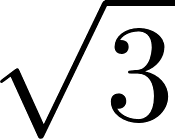when three phase power is used instead of one phase.

### Formulas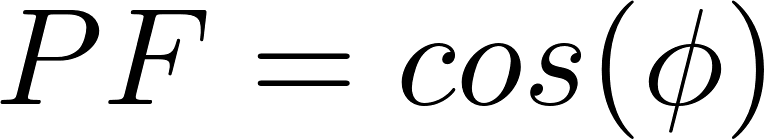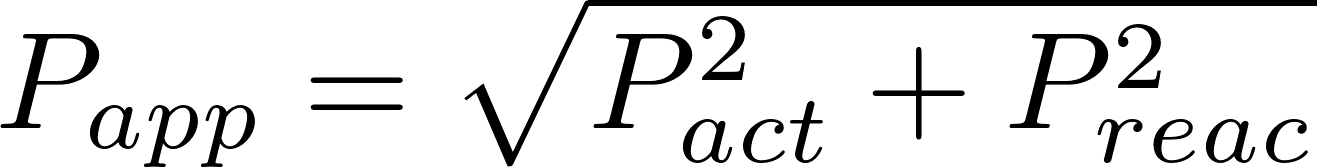#### One phase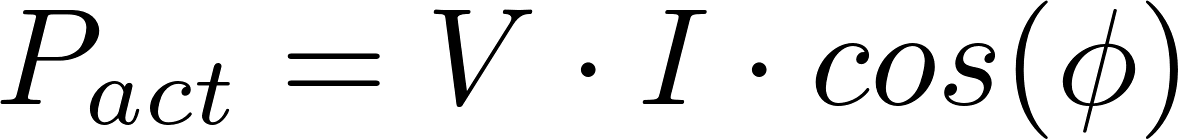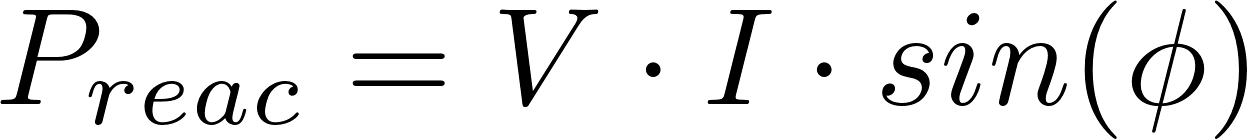#### Three phase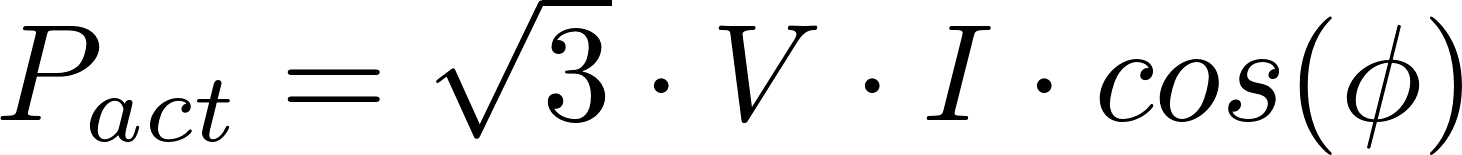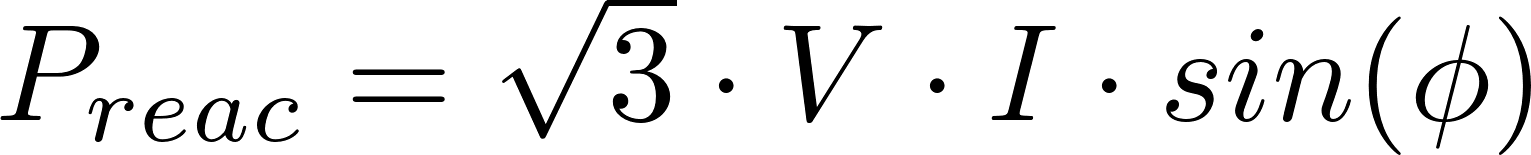PF is the power factor.
P act is the symbol for active power and is measured in watt (W).
P reac is the symbol for reactive power and is measured in volt-ampere reactive (VAR).
P app is the symbol for apparent power and is measured in volt-ampere (VA).
V is the symbol for voltage and is measured in volt (V).
I is the symbol for current and is measured in ampere (A).
φ is the symbol for phase and is measured in degrees.